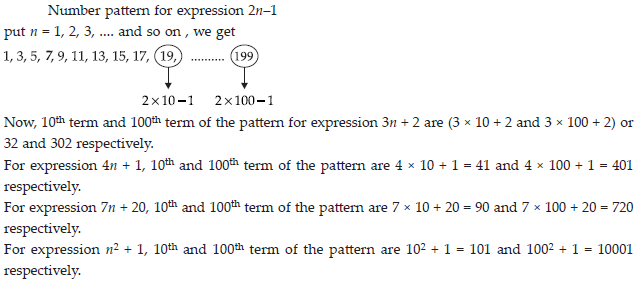Algebraic Expressions (Maths) Class 7 - NCERT Questions
Q 1.

Get the algebraic expressions in the following cases using variables, constants and arithmetic operations.
(i) Subtraction of z from y.
(ii) One-half of the sum of numbers x and y.
(iii) The number z multiplied by itself.
(iv) One-fourth of the product of numbers p and q.
(v) Numbers x and y both squared and added.
(vi) Number 5 added to three times the product of numbers m and n.
(vii) Product of numbers y and z subtracted from 10.
(viii) Sum of numbers a and b subtracted from their product.

SOLUTION: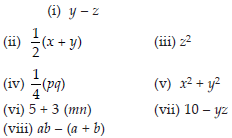Q 2.

(i) Identify the terms and their factors in the following expressions show the terms and factors by tree diagrams.
(A) x – 3 (B) 1 + x + x2
(C) y – y3 (D) 5xy2 + 7x2y
(E) – ab + 2b2 – 3a2
(ii) Identify terms and factors in the expressions given below :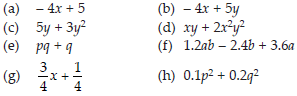SOLUTION: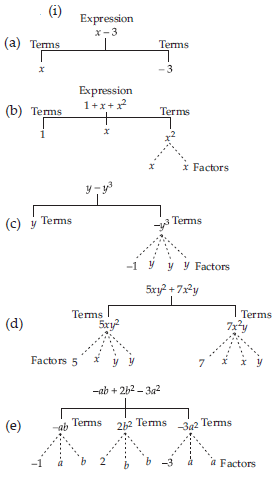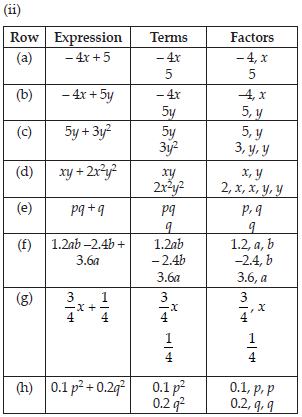Q 3.

Identify the numerical coefficients of terms (other than constants) in the following expressions:
(i) 5 – 3t2
(ii) 1 + t + t2 + t3
(iii) x + 2xy + 3y
(iv) 100 m + 1000 n
(v) –p2q2 + 7pq
(vi) 1.2a + 0.8b
(vii) 3.14 r2
(viii) 2(l + b)
(ix) 0.1y + 0.01y2

SOLUTION: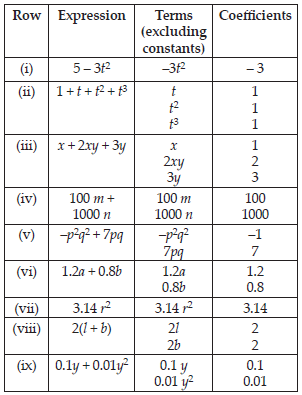Q 4.

(A) Identify terms which contains x and give the coefficient of x.
(i) y2x + y (ii) 13y2 – 8xy
(iii) x + y + 2 (iv) 5 + z + zx
(v) 1 + x + xy (vi) 12xy2 + 25
(vii) 7x + xy2
(B) Identify terms which contains y2 and give the coefficient of y2.
(i) 8 – xy2 (ii) 5y2 + 7x
(iii) 2x2y – 15xy2 + 7y2

SOLUTION: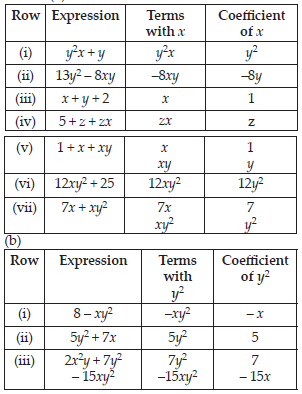Q 5.

Classify into monomials, binomials and trinomials.
(i) 4y – 7z (ii) y2
(iii) x + y – xy (iv) 100
(v) ab – a – b (vi) 5 –3t
(vii) 4p2q –4pq2 (viii) 7mn
(ix) z2 – 3z + 8 (x) a2 + b2
(xi) z2 + z (xii) 1 + x + x2

SOLUTION: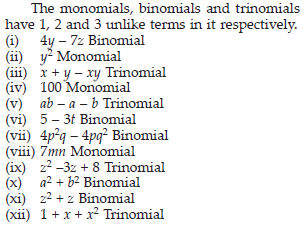Q 6.

State whether a given pair of terms is of like or unlike terms.
(i) 1, 100 (ii) −7x,5/2x,
(iii) –29x, – 29y (iv) 14xy, 42yx
(v) 4m2p, 4mp2 (vi) 12xz, 12x2z2

SOLUTION: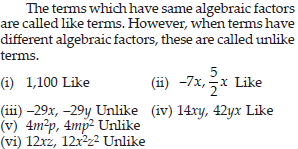Q 7.

Identify like terms in the following:
(A) –xy2, –4yx2, 8x2, 2xy2, 7y, –11x2, –100x, –11yx, 20x2y, –6x2, y, 2xy, 3x
(B) 10pq, 7p, 8q, –p2q2, –7qp, – 100q, –23, 12q2p2, – 5p2, 41, 2405p, 78qp, 13p2q, qp2, 701p2

SOLUTION: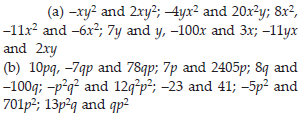Q 8.

Simplify by combining like terms:
(i) 21b – 32 + 7b –20b
(ii) –z2 + 13z2 –5z + 7z2 – 15z
(iii) p – (p – q) –q –(q – p)
(iv) 3a – 2b – ab –(a – b + ab) + 3ab + b – a
(v) 5x2y – 5x2 + 3yx2 –3y2 + x2 – y2 + 8xy2 – 3y2
(vi) (3y2 + 5y – 4) – (8y – y2 – 4)

SOLUTION: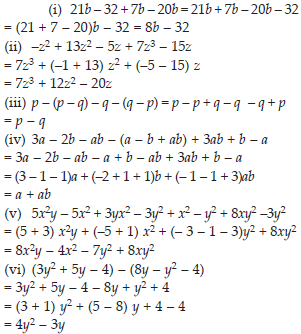Q 9.

(i) 3mn, –5mn, 8mn, –4mn
(ii) t –8tz, 3tz – z, z – t
(iii) –7mn + 5, 12mn + 2, 9mn –8, –2mn – 3
(iv) a + b – 3, b – a + 3, a – b + 3
(v) 14x + 10y – 12xy – 13, 18 – 7x – 10y + 8xy, 4xy
(vi) 5m – 7n, 3n – 4m + 2, 2m – 3mn – 5
(vii) 4x2y, –3xy2, –5xy2, 5x2y
(viii)3p2q2 – 4pq + 5, –10p2q2, 15 + 9pq + 7p2q2
(ix) ab – 4a, 4b – ab, 4a – 4b
(x) x2 – y2 – 1, y2 – 1 – x2, 1 – x2 – y2

SOLUTION: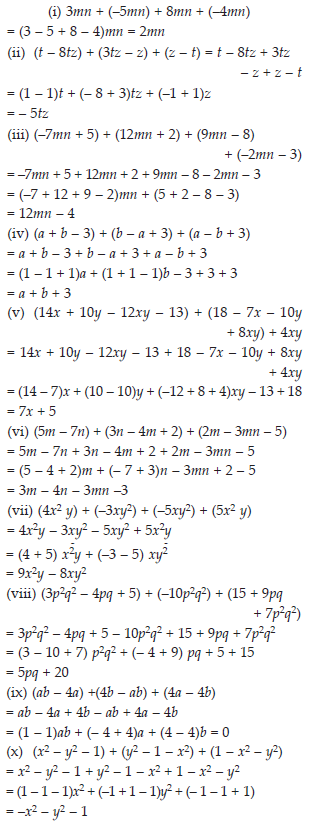Q 10.

Subtract :
(i) –5y2 from y2
(ii) 6xy from –12xy
(iii) (a – b) from (a + b)
(iv) a(b – 5) from b (5 – a)
(v) – m2 + 5mn from 4m2 – 3mn + 8
(vi) – x2 + 10x – 5 from 5x – 10
(vii) 5a2 –7ab + 5b2 from 3ab – 2a2 – 2b2
(viii) 4pq – 5q2 – 3p2 from 5p2 + 3q2 – pq

SOLUTION: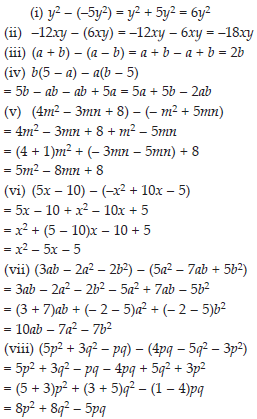Q 11.

(A) What should be added to x2 + xy + y2 to obtain 2x2 + 3xy?
(B) What should be subtracted from 2a + 8b + 10 to get – 3a + 7b + 16?

SOLUTION: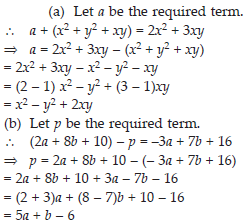Q 12.

What should be taken away from 3x2 – 4y2 + 5xy + 20 to obtain – x2 – y2 + 6xy + 20 ?

SOLUTION: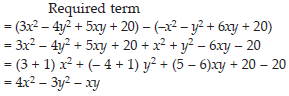Q 13.

(A) From the sum of 3x – y + 11 and –y – 11, subtract 3x – y – 11.
(B) From the sum of 4 + 3x and 5 – 4x + 2x2, subtract the sum of 3x2 – 5x and –x2 + 2x + 5.

SOLUTION: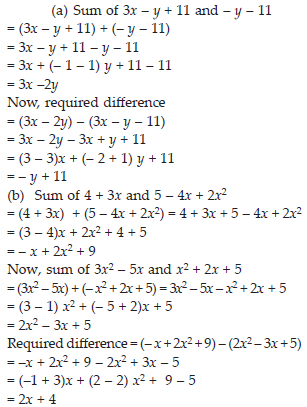Q 14.

If m = 2, find the value of:
(i) m – 2 (ii) 3m – 5
(iii) 9 – 5m (iv) 3m2 – 2m – 7
(v) (5m/2)-4

SOLUTION: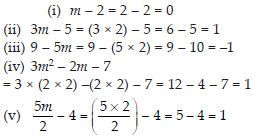Q 15.

If p = –2, find the value of:
(i) 4p + 7 (ii) –3p2 + 4p + 7
(iii) –2p3 – 3p2 + 4p + 7

SOLUTION: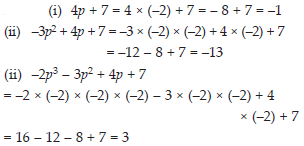Q 16.

Find the value of the following expressions, when x = –1:
(i) 2x – 7 (ii) –x + 2
(iii) x2 + 2x + 1 (iv) 2x2 –x – 2

SOLUTION: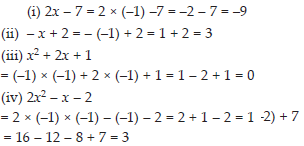Q 17.

If a = 2, b = –2, then find the value of:
(i) a2 + b2 (ii) a2 + ab + b2
(iii) a2 – b2

SOLUTION: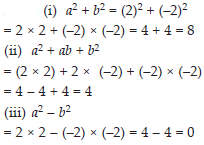Q 18.

When a = 0, b = –1, find the value of the given expressions:
(i) 2a + 2b (ii) 2a2 + b2 + 1
(iii) 2a2b + 2ab2 + ab (iv) a2 + ab + 2

SOLUTION: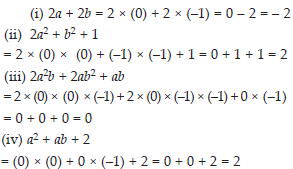Q 19.

Simplify the expressions and find the value, if x is equal to 2
(i) x + 7 + 4 (x – 5)
(ii) 3(x + 2) + 5x – 7
(iii) 6x + 5(x –2)
(iv) 4(2x – 1) + 3x + 11

SOLUTION: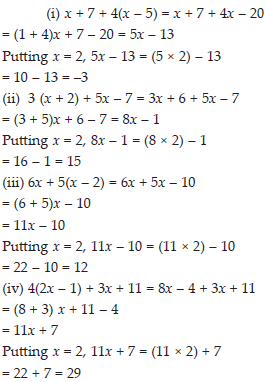Q 20.

Simplify these expressions and find their values if x = 3, a = –1, b = –2.
(i) 3x – 5 – x + 9 (ii) 2 – 8x + 4x + 4
(iii) 3a + 5 – 8a + 1 (iv) 10 – 3b – 4 – 5b
(v) 2a – 2b – 4 – 5 + a

SOLUTION: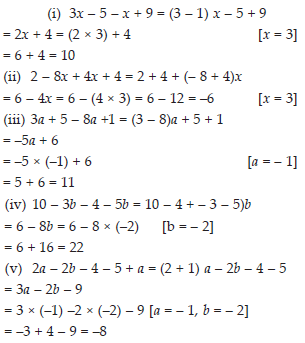Q 21.

(i) If z = 10, find the value of z3 – 3(z – 10).
(ii) If p = –10, find the value of p2 – 2p –100.

SOLUTION: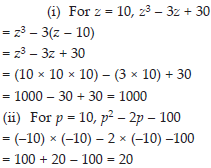Q 22.

What should be the value of a if the value of 2x2 + x – a equals to 5, when x = 0?

SOLUTION: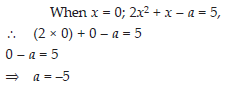Q 23.

Simplify the expression 2(a2 + ab) + 3 – ab and find its value when a = 5 and b = –3.

SOLUTION: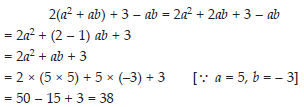Q 24.

Observe the patterns of digits made from line segments of equal length. You will find such segmented digits on the display of electronic watches or calculators.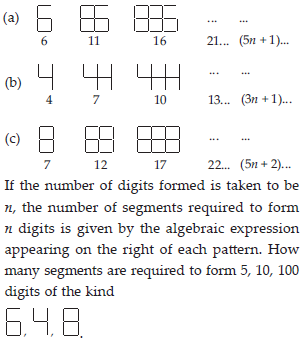SOLUTION: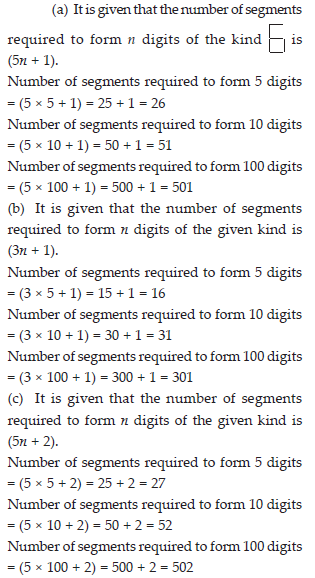Q 25.

Use the given algebraic expression to complete the table of number patterns.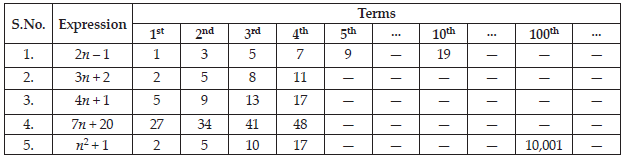SOLUTION: